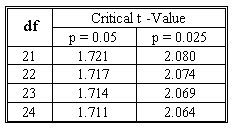### CFA Practice Question

There are 208 practice questions for this study session.

### CFA Practice Question

You have obtained the following sample regression equation from 25 samples:

y-hatt = 2.304 + 3.412 Xt1 - 4.657 X2t

The estimated standard errors are 0.87, 1.23 and 1.78 for b0, b1 and b2, respectively. Construct a 95% confidence interval for β2.